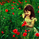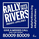2580 views
2580
MACD Overlay indicator plots the MACD directly on price. This helps you better understand the MACD indicator and filter the false signals.Sir, How do you color the bars, with an MTF option, thank youamazing
how can i set this setting i don't know to about set codeRiteish021
@Riteish021, If you are refering to MACD code in the above comment, just copy and paste it in blank indicator script, save it and your indicator is ready.Kumaran1964
@Kumaran1964, This is the code for MACD Indicator:

//@version=2
study("MACD")
src=input(close, title="Field")
s=input(26, minval=1, title="Slow")
f=input(12, minval=1, title="Fast")
si=input(9, minval=1, title="Signal")

fast=ema(src, f)
slow=ema(src, s)

macd=fast-slow
signal=sma(macd, si)
hist=macd-signal

pos_hist=max(0, hist)
neg_hist=min(0, hist)

plot(pos_hist, color=pos_hist>pos_hist?green:red, style=histogram, linewidth=3, title="Positive Histogram")
plot(neg_hist, color=neg_hist>neg_hist?green:red, style=histogram, linewidth=3, title="Negative Histogram")
p1=plot(macd, color=macd>macd?green:red, style=line, linewidth=2, title="MACD")
p2=plot(signal, color=signal>signal?green:red, style=line, linewidth=2, title="Signal")
fill(p1, p2, color=macd>signal ? green:red, title="FILL", transp=50)

ma_len=input(89, title="SMA")
ma=sma(macd, ma_len)

plot(ma, title="SMA", color=ma>ma?green:red, style=line, linewidth=1)